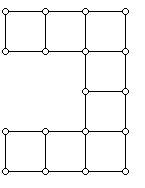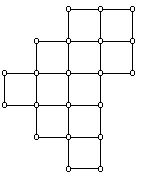A Sampler of Mathematics Research Problems for Undergraduates and High School Students

prepared by:

Joseph Malkevitch
Department of Mathematics
York College (CUNY)
Jamaica, New York 11451

Email: malkevitch@york.cuny.edu (for additions, suggestions, and corrections)

Problem 1:

Determine the number of inequivalent nets for an r x s x t box. A net is a plane polygon which can be folded to form a polyhedron. It is known that there are 11 nets for the 1 x 1 x 1 box, the cube. An example of one such net is shown below:To start, you might try to find all the inequivalent nets of a 1 x 1 x 2 box. The polygon for this net would involve two 1 x 1 squares and four 1 x 2 rectangles.

It is still an unsolved problem to show that there is a way to cut along the edges of every convex 3-dimensional polyhedron and fold out the result to form a flat polygon in the plane where the faces of the original polyhedron do not overlap.

Problem 2:

Any plane simple polygon can triangulated. This means that one adds non-intersecting diagonals to the polygon so all the regions of the polygon become triangles. In the diagram below the original seven sided polygon (n = 7) is shown with think black lines. The diagonals which have been added are shown with thin lines. At each vertex of the polygon a number is shown, which gives the number of line segments which meet at that particular vertex. (In terms of graph theory, the number shown is the valence or degree of that vertex of the diagram, interpreted as a graph.) For the example shown, the set of numbers obtained in this way is: { 2, 2, 3, 3, 3, 3, 6 }. The problem is to determine given a set D of positive integers, when is D the set of numbers that can be obtained from some simple polygon which has been triangulated.Problem 3:

A polyomino is a plane figure created by pasting a collection of 1 x 1 unit squares together along edges. A typical 8-omino, consisting of 8 such squares is shown below:A polyomino W is called vertically convex if every vertical line cutting the interior of a square intersects W in a connected collection squares. A polyomino W is called horizontally convex if every horizontal cutting the interior of a square intersects W in a connected collection of squares. A polyomino W which is both vertically and horizontally convex will be called convex. The polyomino above is horizontally convex but not vertically convex. A polyomino is called L-convex if for any pair of square in the polyomino there is a path with at most one bend joining these two squares. The polyomino X below is a convex 12-omino. This polyomino is L-convex.The polyomino Y (it is an 11-omino) below is convex but not L convex. In particular, the first cell in the third row and the second cell in the top row can not be joined by a path with a single bend.The polyomino X can be thought of as consisting of rows with 3, 3, 3, 2, and 1 squares.

Problem 4:

Determine which sequences of positive integers (the example X above being the sequence 3, 3, 3, 2, 1) give rise to a convex polyomino with rows having the number of squares in the given sequence. For example, given the sequence 1, 4, 5, 2 one could use the polyomino obtained from X by rotating the diagram shown by 90 degrees in the clockwise direction. The problem can be repeated for L-convex polyominoes.

Problem 5:

a. Which convex polyominoes will tile a rectangle?

b. Which convex polyominoes will tile the plane?

c. Which L-convex polyominoes will tile a rectangle?

d. Which L-convex polyominoes will tile the plane?

Note: A good place to start with these questions is to see if you can find examples for each of the 4 cases where the type of polyomino described will not be able to tile in the described way. If such examples exist, then one can try to find out what additional requirements on the polyomino might make a tiling possible.